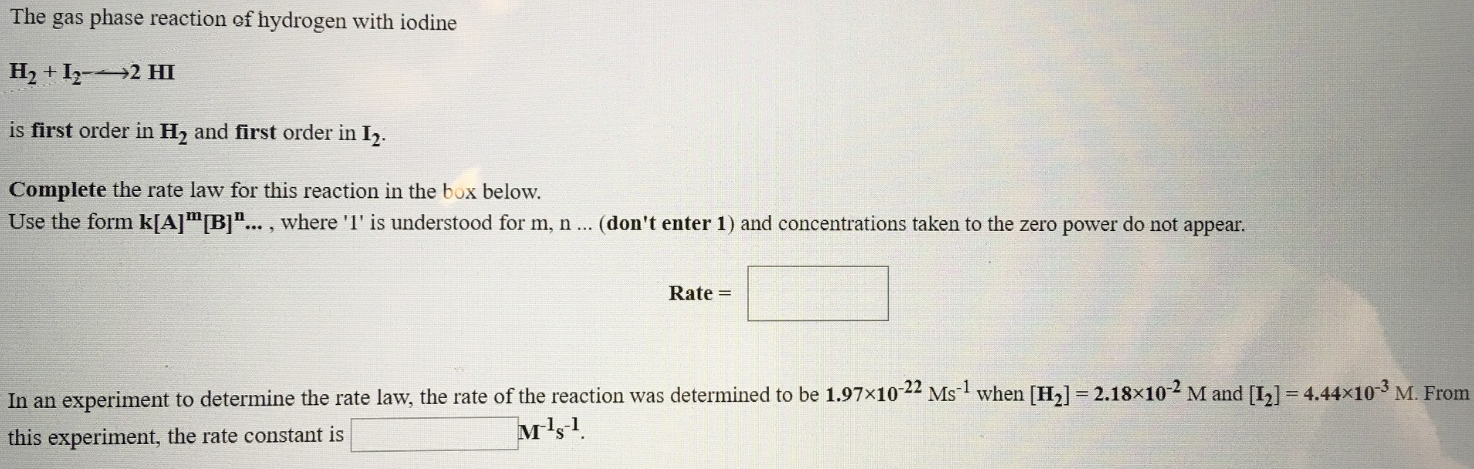# The gas phase reaction of hydrogen with iodine H2 + I2 → 2 HI is first order in H2 and first order in I2. Complete the rate law for this reaction in the box below. Use form k[A] ^m [B] ^n..., where "1" is understood for m, n ... (don't enter 1 ) and concentrations taken to the zero power do not appear. In an experiment to determine the rate law, the rate of the reaction was determined to be 1.97x10^-22 Ms^-1 when [H2] = 2.18x10^-2 M and [I2] = 4.44x10^-3 M. From this experiment, the rate constant is ________ M^-1s^-1.